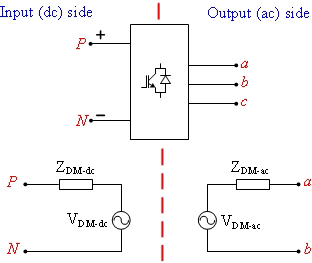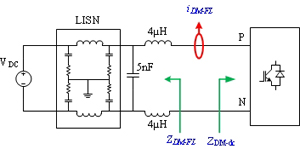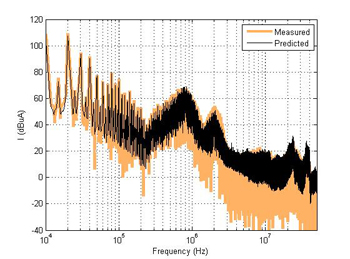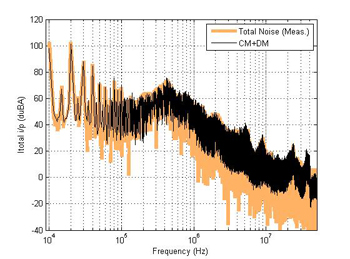LIBRARY

# EMI Terminal Modelling of DC-Fed Motor Drives II: Differential Mode and Total Noise

Year: 2013Fig. 1. DM noise models for a motor-drive system.
A three-terminal noise-model was shown earlier to predict the common-mode (CM) currents at the input and output side of a dc fed motor-drive system. Accuracy of this model was found to be good up to 30 MHz. The DO-160 standards for aerospace applications however are based on total noise, therefore having just a CM model is not enough when simulating for EMC compliance. This paper proposes simple terminal models to predict the differential mode (DM) noise currents in a motor-drive system. In the end, predictions from the CM and DM models are combined to estimate the total noise at the input and output side of the motor-drive system.

The model is based on Thevenin equivalent of a two terminal network. Investigations re-vealed that in differential mode (DM), the input and output side of the motor-drive can be considered decoupled, provided the dc-link at the input side is adequately decoupled. Under this assumption the DM model can be split and extracted independently for both input and output side. This idea is shown in Fig. 1.

The model impedances (ZDM-dc and ZDM-ac) are approximated using direct measurements under power-off conditions and the model sources (VDM-dc and VDM-ac) are extracted experimentally using terminal modelling techniques. Once the models are extracted, the prediction of DM EMI currents for an input side DM filter shown in Fig. 2 is carried out. Also, the total noise is estimated by adding the predicted noise from the DM model and the CM noise from the previously developed CM model. These prediction results are shown in Fig. 3 and 4.Fig. 2. DM noise models for a motor-drive system.Fig. 3. DM noise prediction (iDM-FL).Fig. 4. Total-noise prediction at input side.